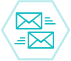# Sample size requirements for knowledge-based treatment planning

To determine how training set size affects the accuracy of knowledge-based treatment planning (KBP) models.

The authors selected four models from three classes of KBP approaches, corresponding to three distinct quantities that KBP models may predict: dose-volume histogram (DVH) points, DVH curves, and objective function weights. DVH point prediction is done using the best plan from a database of similar clinical plans; DVH curve prediction employs principal component analysis and multiple linear regression; and objective function weights uses either logistic regression or K-nearest neighbors. The authors trained each KBP model using training sets of sizes n = 10, 20, 30, 50, 75, 100, 150, and 200. The authors set aside 100 randomly selected patients from their cohort of 315 prostate cancer patients from Princess Margaret Cancer Center to serve as a validation set for all experiments. For each value of n, the authors randomly selected 100 different training sets with replacement from the remaining 215 patients. Each of the 100 training sets was used to train a model for each value of n and for each KBT approach. To evaluate the models, the authors predicted the KBP endpoints for each of the 100 patients in the validation set. To estimate the minimum required sample size, the authors used statistical testing to determine if the median error for each sample size from 10 to 150 is equal to the median error for the maximum sample size of 200.

The minimum required sample size was different for each model. The DVH point prediction method predicts two dose metrics for the bladder and two for the rectum. The authors found that more than 200 samples were required to achieve consistent model predictions for all four metrics. For DVH curve prediction, the authors found that at least 75 samples were needed to accurately predict the bladder DVH, while only 20 samples were needed to predict the rectum DVH. Finally, for objective function weight prediction, at least 10 samples were needed to train the logistic regression model, while at least 150 samples were required to train the K-nearest neighbor methodology.

In conclusion, the minimum required sample size needed to accurately train KBP models for prostate cancer depends on the specific model and endpoint to be predicted. The authors' results may provide a lower bound for more complicated tumor sites.

Medical physics. 2016 Mar [Epub]

Justin J Boutilier, Tim Craig, Michael B Sharpe, Timothy C Y Chan